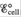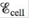# Consider the galvanic cell based on the following half-reactions: a. Determine the overall cell reaction and calculate b. Calculate ΔG ° and K for the cell reaction at 25°C. c. Calculate at 25°C when [Zn 2+ ] = 0.10 M and [Fe 2+ ] = 1.0 × 10 −5 M.### Chemistry: An Atoms First Approach

2nd Edition
Steven S. Zumdahl + 1 other
Publisher: Cengage Learning
ISBN: 9781305079243

#### Solutions

Chapter
Section### Chemistry: An Atoms First Approach

2nd Edition
Steven S. Zumdahl + 1 other
Publisher: Cengage Learning
ISBN: 9781305079243
Chapter 17, Problem 77E
Textbook Problem
1 views

## Consider the galvanic cell based on the following half-reactions:a. Determine the overall cell reaction and calculateb. Calculate ΔG° and K for the cell reaction at 25°C.c. Calculateat 25°C when [Zn2+] = 0.10 M and [Fe2+] = 1.0 × 10−5 M.

(a)

Interpretation Introduction

Interpretation:

The half-reaction taking place in a galvanic cell is given. Various questions based on the cell reaction are to be answered.

Concept introduction:

The relationship between reduction potential and standard reduction potential value and activities of species present in an electrochemical cell at a given temperature is given by the Nernst equation.

The value of Ecell is calculated using Nernst formula,

E=E°(RTnF)ln(Q)

At room temperature the above equation is specifies as,

E=E°(0.0591n)log(Q)

This relation is further used to determine the relation between ΔG° and K , ΔG° and E°cell.

To determine: The overall cell reaction and E°cell for the given cell reaction.

### Explanation of Solution

Explanation

The reaction taking place on cathode,

Fe2++2eFeE°red=0.44V

The reaction taking place at anode,

ZnZn2++2eE°ox=0.76V

Add both the reduction half and oxidation half-reaction.

Fe2++2eFeZnZn2++2e

The overall cell reaction is,

Zn+

(b)

Interpretation Introduction

Interpretation:

The half-reaction taking place in a galvanic cell is given. Various questions based on the cell reaction are to be answered.

Concept introduction:

The relationship between reduction potential and standard reduction potential value and activities of species present in an electrochemical cell at a given temperature is given by the Nernst equation.

The value of Ecell is calculated using Nernst formula,

E=E°(RTnF)ln(Q)

At room temperature the above equation is specifies as,

E=E°(0.0591n)log(Q)

This relation is further used to determine the relation between ΔG° and K , ΔG° and E°cell.

To determine: The value of ΔG° and K for the cell reaction at 25°C.

(c)

Interpretation Introduction

Interpretation:

The half-reaction taking place in a galvanic cell is given. Various questions based on the cell reaction are to be answered.

Concept introduction:

The relationship between reduction potential and standard reduction potential value and activities of species present in an electrochemical cell at a given temperature is given by the Nernst equation.

The value of Ecell is calculated using Nernst formula,

E=E°(RTnF)ln(Q)

At room temperature the above equation is specifies as,

E=E°(0.0591n)log(Q)

This relation is further used to determine the relation between ΔG° and K , ΔG° and E°cell.

To determine: The value of Ecell for the given concentrations of Zn2+ and Fe2+.

### Still sussing out bartleby?

Check out a sample textbook solution.

See a sample solution

#### The Solution to Your Study Problems

Bartleby provides explanations to thousands of textbook problems written by our experts, many with advanced degrees!

Get Started

Find more solutions based on key concepts
Which of the following foods is fare) rich in beta-carotene? sweet potatoes pumpkin spinach all of the above

Nutrition: Concepts and Controversies - Standalone book (MindTap Course List)

Where should the police car in Figure 6-9 have parked to make a good measurement?

Horizons: Exploring the Universe (MindTap Course List)

In which domain and kingdom are humans classified?

Biology: The Dynamic Science (MindTap Course List)

Match the term listed in Column A with its definition from Column B

Nutrition Through the Life Cycle (MindTap Course List)

Example 10.6 Angular Acceleration of a Wheel A wheel of radius R mass M and moment of inertia I is mounted on a...

Physics for Scientists and Engineers, Technology Update (No access codes included)